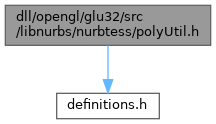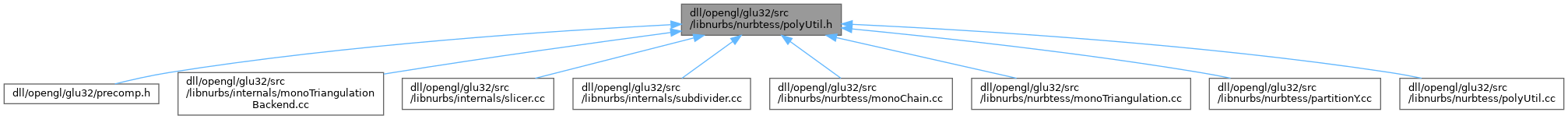ReactOS 0.4.15-dev-6068-g8061a6f
polyUtil.h File Reference
`#include "definitions.h"`
Include dependency graph for polyUtil.h:This graph shows which files directly or indirectly include this file:Go to the source code of this file.

## Functions

Real area (Real A, Real B, Real C)

Int pointLeftLine (Real A, Real B, Real P)

Int pointLeft2Lines (Real A, Real B, Real C, Real P)

## ◆ area()

 Real area ( Real A, Real B, Real C )

Definition at line 43 of file polyUtil.cc.

44{
45 Real Bx, By, Cx, Cy;
46 Bx = B - A;
47 By = B - A;
48 Cx = C - A;
49 Cy = C - A;
50 return Bx*Cy - Cx*By;
51
52/* return (B-A)*(C-A) - (C-A)*(B-A);*/
53}
Definition: ehthrow.cxx:93
Definition: ehthrow.cxx:54
Definition: terminate.cpp:24
float Real
Definition: definitions.h:36

Referenced by pointLeft2Lines(), and pointLeftLine().

## ◆ pointLeft2Lines()

 Int pointLeft2Lines ( Real A, Real B, Real C, Real P )

Definition at line 78 of file polyUtil.cc.

79{
80 Int C_left_AB = (area(A, B, C)>0);
81 Int P_left_AB = (area(A, B, P)>0);
82 Int P_left_BC = (area(B, C, P)>0);
83
84 if(C_left_AB)
85 {
86 return (P_left_AB && P_left_BC);
87 }
88 else
89 return (P_left_AB || P_left_BC);
90}
int Int
Definition: definitions.h:37
#define P(row, col)
Real area(Real A, Real B, Real C)
Definition: polyUtil.cc:43

Referenced by MC_partitionY(), and partitionY().

## ◆ pointLeftLine()

 Int pointLeftLine ( Real A, Real B, Real P )

Definition at line 64 of file polyUtil.cc.

65{
66 if(area(A, B, P) >0) return 1;
67 else return 0;
68}